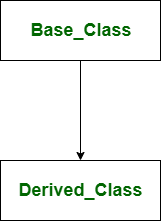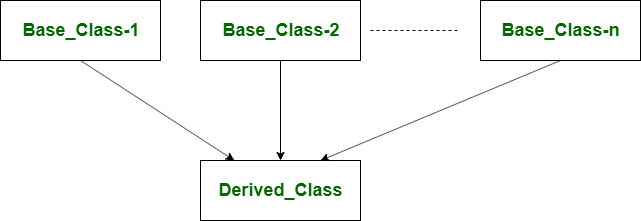# Difference between Single and Multiple Inheritance in C++

• Last Updated : 12 Jun, 2022

Single Inheritance: Single inheritance is one in which the derived class inherits the single base class either publicly, privately or protectedly. In single inheritance, the derived class uses the features or members of the single base class. These base class members can be accessed by derived class or child class according to the access specifier specified during inheriting the parent class or base class.Syntax:

```Class DerivedClass_name : access_specifier Base_Class
{
//Class's Body
}; ```

Example of Single Inheritance:

## CPP

 `#include``using` `namespace` `std;``class` `A {``public``:``    ``int` `k = 1000;``    ``float` `salary = 80000;``};``class` `B : ``public` `A {``public``:``    ``float` `bonus = 8000;``    ``void` `ts()``    ``{``        ``cout<<"Total salary.."<<(salary + bonus)<

Output:

```Salary: 80000
Bonus: 8000
total salary..88000 ```

In the this example, A is the base class or parent class and B is the derived class or child class. Multiple Inheritance: Multiple inheritance is one in which the derived class acquires two or more base classes. In multiple inheritance, the derived class are allowed to use the joint features of the inherited base classes. Every base class is inherited by the derived class by notifying the separate access specifier for each of them. The base class members can be accessed by derived class or child class according to the access specifier specified during inheriting the parent class or base class.## C++

 `// Example:` `#include``using` `namespace` `std;` `class` `A``{``    ``protected``:``    ``int` `a;``    ``public``:``        ``void` `set_A()``        ``{``            ``cout<<``"Enter the Value of A="``;``            ``cin>>a;``            ` `        ``}``        ``void` `disp_A()``        ``{``            ``cout<

Output

```Enter the Value of A= 5
5
Enter the Value of B= 9
9

Product of 5 * 9 = 45```

## C++

 `// Example:``#include``using` `namespace` `std;` `class` `A``{``    ``protected``:``    ``int` `a;``    ``public``:``        ``void` `set_A(``int` `x)``        ``{``             ``a=x;           ``        ``}``        ``void` `disp_A()``        ``{``            ``cout<

Output

`Product of 4 * 5 = 20`

Syntax:

```Class DerivedClass_name : access_specifier Base_Class1, access_specifier Base_Class2
{
//Class's Body
}; ```

Example of Multiple Inheritance:

## CPP

 `#include``using` `namespace` `std;``class` `A {``public``:``    ``float` `salary = 80000;``};` `class` `B {``public``:``    ``float` `bonus = 8000;``};` `class` `C : ``public` `A, ``public` `B {``public``:``    ``void` `ts()``    ``{``        ``cout<<"Total salary.."<<(salary + bonus)<

Output:

`Total salary..88000 `

In the this example, A and B are the base classes and C is the derived class.

## C++

 `// Example:` `#include  ``using` `namespace` `std; ``class` `A ``{ ``    ``protected``: ``     ``int` `a; ``    ``public``: ``    ``void` `get_a(``int` `n) ``    ``{ ``        ``a = n; ``    ``} ``}; ``  `  `class` `B ``{ ``    ``protected``: ``    ``int` `b; ``    ``public``: ``    ``void` `get_b(``int` `n) ``    ``{ ``        ``b = n; ``    ``} ``}; ` `class` `C : ``public` `A,``public` `B ``{ ``   ``public``: ``    ``void` `display() ``    ``{ ``        ``cout << ``"The value of a is : "` `<

Output

```The value of a is : 10
The value of b is : 20
Product of a and b is : 200```

Difference between Single and multiple Inheritance:

My Personal Notes arrow_drop_up